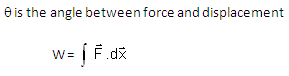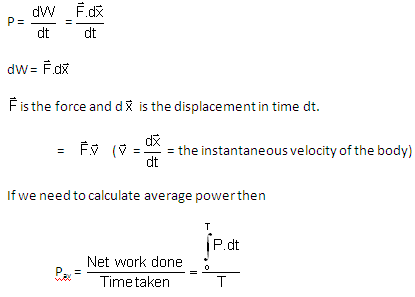# Work Power Energy Assignment Help, Homework Help, Physics Help

### Physics Assignment Help >> Work, Power and Energy, Energy Conservation We at ExpertsMind offer online physics solutions for work, power and energy. We offer email based assignment help, homework help and project help services for students who need quick help in their course preparation and college and university exam preparation. We provide help with online qualified physics experts who have ability to solve your toughest problems in work, power and energy physics topics. We cover complete syllabus of work, energy and power, kinetic and potential energy, energy conservation and its application, different forms of energy, elastic collision in one and two dimensions. We provide work, power and energy assignment help from high school level to college level.

Theory of Work, Power and Energy

### Work is defined by the dot product of force and displacementWhere F = force active on a point, S = Displacement andPower is defined by the rate at which work is done.Energy is defined as capacity to do work. There are two types of mechanical energy

a. Kinetic Energy
b. Potential Energy

Kinetic Energy: It is defined as energy possessed by a body by its motion. If mass of body is m and speed is v then kinetic energy

K.E. = 1/2 mv2

Potential Energy is defined as the energy possessed by the body by its position and strain.

It can be defined for conservative force field only. In case of uniform gravity, potential energy is mgh m m=mass of body

h= height from reference level

Unit and Dimensions

Energy and work have same units. Power is measured at the rate at which work is done.Related Topics: Work, Power, Energy, Unit and Dimensions, Conversation between different systems of units, Potential Energy, Kinetic Energy, Work Kinetic Energy Theorem, Conservation of Energy, Motion in a Vertical Circle, Critical Velocity, Collisions, Elastic Collision, Inelastic Collision, Concept of Coefficient of restitution

# Why Us ?